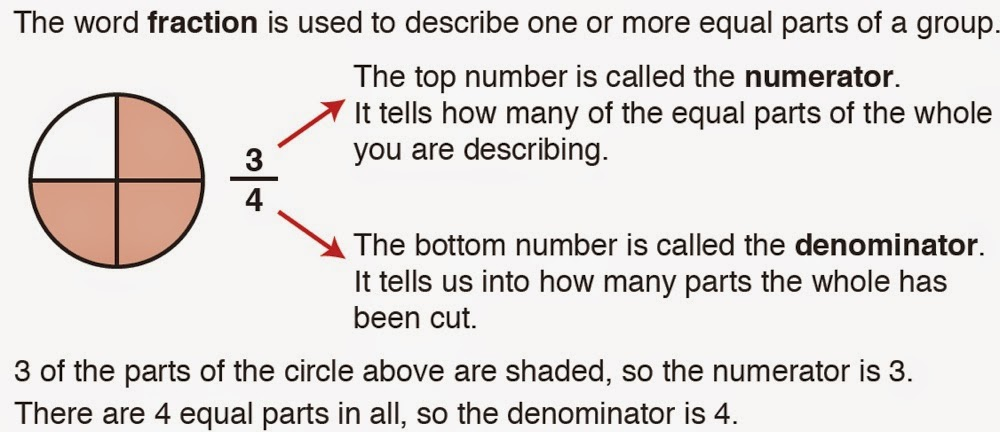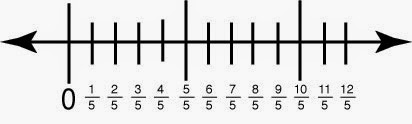## Tuesday, November 25, 2014

### Excel Math: Calming the Frenzy Over FractionsExcel Math Lesson on Fractions
Fractions have always been a nemesis for students and teachers alike.

Now with new standards, the emphasis on fractions is even greater than before.

Students are expected to master fractions sooner than ever, and Excel Math is here to help!

In Excel Math lessons, fractions are introduced in clear and concise language, starting with Grade 1 and continuing in complexity through Grade 6.

See an example of an Excel Math lesson on fractions above.

Following the brief lesson, students are given a chance to work with fractions while the teacher is nearby, ready to help those who need assistance. Fractions on a number line are now introduced to give students a visual image of how fractions are ordered.Fractions on a Number Line

Consumable pages give students lots of opportunities to practice the math problems.

Excel Math's unique CheckAnswer system lets students self assess so they can discover and correct most mistakes on their own.

During Guided Practice, students can call on a teacher to help them when they run into difficulty.After two weeks of practice, fractions are included as homework. A week or two later, fractions finally show up on the weekly test.

By this time, students are very familiar with fractions.

Repetition helps get fractions into long-term memory, which builds student confidence and success in math — including success on these new assessments.

As a result, Excel Math students are testing off the charts in mathematics.

You may also enjoy these articles:

Excel Math Helps Students Raise Test Scores

Math Placement Tests: Off to a Great Start

Five Steps to Solving Word Problems

Common Core—A New View on Teaching Math